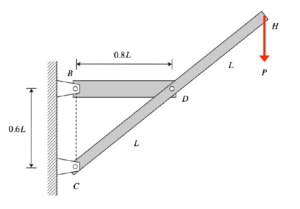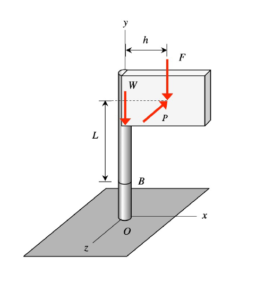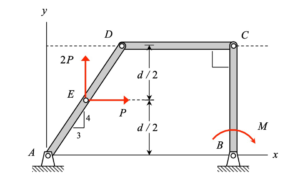# HOMEWORK 30.APlease post questions here on the homework. And, take time to answer questions posted by others. You can learn both ways.

# HOMEWORK 30.BPlease post questions here on the homework. And, take time to answer questions posted by others. You can learn both ways.

# HOMEWORK 29.APlease post questions here on the homework. And, take time to answer questions posted by others. You can learn both ways.

# HOMEWORK 29.BPlease post questions here on the homework. And, take time to answer questions posted by others. You can learn both ways.

# HOMEWORK 28.BPlease post questions here on the homework. And, take time to answer questions posted by others. You can learn both ways.

# HOMEWORK 28.APlease post questions here on the homework. And, take time to answer questions posted by others. You can learn both ways.

# HOMEWORK 26.APlease post questions here on the homework. And, take time to answer questions posted by others. You can learn both ways.

# HOMEWORK 26.BPlease post questions here on the homework. And, take time to answer questions posted by others. You can learn both ways.

# HOMEWORK 25.BPlease post questions here on the homework. And, take time to answer questions posted by others. You can learn both ways.

# HOMEWORK 25.APlease post questions here on the homework. And, take time to answer questions posted by others. You can learn both ways.